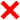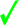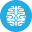# PHP Language | 10 Minute‐Test 18

Instruction

• Total number of questions : 10.
• Time alloted : 10 minutes.
• Each question carry 1 mark.
• No Negative marks
• DO NOT refresh the page.
• All the best :-).

1.

Which keyword is used to refer to properties or methods within the class itself?

A.
 privateB.
 publicC.
 protectedD.
 \$thisCorrect Answer: Option D

Explanation:

None.

2.

Which feature allows us to call more than one method or function of the class in single instruction?

A.
 TypecastingB.
 Method IncludingC.
 Method addingD.
 Method chainingCorrect Answer: Option D

Explanation:

Following is a basic example of method chaining in php: \$a = new Order(); \$a->CreateOrder()->sendOrderEmail()->createShipment();

3.

Which logging option’s description is, if an error occurs when writing to the syslog, send output to the system console?

A.
 LOG_CONSB.
 LOG_NDELAYC.
 LOG_ODELAYD.
 LOG_PERRORCorrect Answer: Option A

Explanation:

None.

4.

Which of the following is/are not an exception?
iii) LogicException
iv) DomainException

A.
 All of the mentionedB.
 Only iv)C.
 iii) and iv)D.
 None of the mentionedCorrect Answer: Option D

Explanation:

None.

5.

What will be the output of the following PHP code?

<?php

\$value = 'car';

\$result = filter_var(\$value, FILTER_VALIDATE_BOOLEAN, FILTER_NULL_ON_FAILURE);

?>

A.
 FALSEB.
 TRUEC.
 NULLD.
 ERRORCorrect Answer: Option C

Explanation:

There is an undocumented filter flag for FILTER_VALIDATE_BOOLEAN. The documentation implies that it will return NULL if the value doesn’t match the allowed true/false values. However this doesn’t happen unless you give it the FILTER_NULL_ON_FAILURE flag.

6.

Which of the following would be a potential match for the Perl-based regular expression /fo{2,4}/ ?
i) fol
ii) fool
iii) fooool
iv) fooooool

A.
 Only i)B.
 ii) and iii)C.
 i), iii) and iv)D.
 i) and iv)Correct Answer: Option B

Explanation:

This matches f followed by two to four occurrences of o.

7.

Which one of the following functions finds the last occurrence of a string, returning its numerical position?

A.
 strlastpos()B.
 strpos()C.
 strlast()D.
 strrpos()Correct Answer: Option D

Explanation:

None

8.

Which function is useful when you want to output the executed command’s result?

A.
 out_cmm()B.
 out_system()C.
 cmm()D.
 system()Correct Answer: Option D

Explanation:

None

9.

Say you want to calculate the date 45 days from the present date which one of the following statement will you use?

A.
 totime(“+45”)B.
 totime(“+45 days”)C.
 strtotime(“+45 days”)D.
 strtotime(“-45 days”)Correct Answer: Option C

Explanation:

The strtotime() function and GNU date syntax is used to calculating the date x days from the present date.

10.

Which of the following statements can be used to add two months to the existing date?

A.
 \$date->modify(‘+2 months’B.
 \$date = modify(‘+2 months’);C.
 \$date = modify(‘2+ months’);D.
 \$date->modify(‘2+ months’);Correct Answer: Option A

Explanation:

To change the date stored by a DateTime object after it has been created, you use DateTime::modify() with a natural language expression.

Try More: Boost your Knowledge
#####Test 27

Submit your test now to view the Results and Statistics with answer explanation.